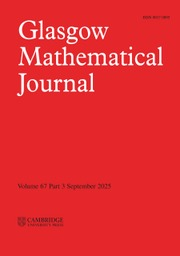Home
Hostname: page-component-7ccbd9845f-6pjjk Total loading time: 0.808 Render date: 2023-01-27T08:59:13.968Z Has data issue: true Feature Flags: { "useRatesEcommerce": false } hasContentIssue trueGlasgow Mathematical Journal

## Abstract

Using Cohen’s classification of symplectic reflection groups, we prove that the parabolic subgroups, that is, stabilizer subgroups, of a finite symplectic reflection group, are themselves symplectic reflection groups. This is the symplectic analog of Steinberg’s Theorem for complex reflection groups.

Using computational results required in the proof, we show the nonexistence of symplectic resolutions for symplectic quotient singularities corresponding to three exceptional symplectic reflection groups, thus reducing further the number of cases for which the existence question remains open.

Another immediate consequence of our result is that the singular locus of the symplectic quotient singularity associated to a symplectic reflection group is pure of codimension two.

Type
Research Article

## Access options

Get access to the full version of this content by using one of the access options below. (Log in options will check for institutional or personal access. Content may require purchase if you do not have access.)

## References

Bosma, W., Cannon, J. and Playoust, C., The Magma algebra system. I. The user language, J. Symb. Comput. 24(3-4) (1997), 235265, Computational algebra and number theory (London, 1993).CrossRefGoogle Scholar
Bellamy, G., On singular Calogero–Moser spaces, Bull. Lond. Math. Soc. 41(2) (2009), 315326.CrossRefGoogle Scholar
Brown, K. A. and Gordon, I., Poisson orders, symplectic reflection algebras and representation theory, J. Reine Angew. Math. 559(559) (2003), 193216.Google Scholar
Bourbaki, N., Groupes et algébres de Lie (Hermann, Paris, 1968).Google Scholar
Bellamy, G. and Schedler, T., A new linear quotient of C$^{4}$ admitting a symplectic resolution, Z. Math 273 (3-4)(2013), 753769.CrossRefGoogle Scholar
Bellamy, G. and Schedler, T., On the (non)existence of symplectic resolutions of linear quotients, Math. Res. Lett. 23(6) (2016), 15371564.CrossRefGoogle Scholar
Bellamy, G., Schmitt, J. and Thiel, U., Towards the classification of symplectic linear quotient singularities admitting a symplectic resolution, Math. Z. To appear.Google Scholar
Cohen, A. M., Finite complex reflection groups, Annales scientifiques de l’ É.N.S. 4th ser. 9(3) (1976), 379436.Google Scholar
Cohen, A. M., Finite quaternionic reflection groups, J. Algebra 64(2) (1980), 293324.CrossRefGoogle Scholar
Etingof, P. and Ginzburg, V., Symplectic refiection algebras, Calogero–Moser space, and deformed Harish–Chandra homomorphism, Invent. Math. 147(2) (2002), 243348.CrossRefGoogle Scholar
Fu, B., A survey on symplectic singularities and symplectic resolutions, Ann. Math. Blaise Pascal 13(2) (2006), 209236.CrossRefGoogle Scholar
GAP, Groups, Algorithms, and Programming, Version 4.11.1. The GAP Group (2021). Available at https://www.gap-system.org.Google Scholar
Ginzburg, V. and Kaledin, D., Poisson deformations of symplectic quotient singularities, Adv. Math. 186(1) (2004), 157.CrossRefGoogle Scholar
Gordon, I., Baby Verma modules for rational Cherednik algebras, Bull. London Math. Soc. 35(03) (2003), 321336.CrossRefGoogle Scholar
Gordeev, N. L., Finite linear groups whose algebra of invariants is a complete intersection, Izv. Akad. Nauk SSSR Ser. Mat. 50(2) (1986), 343392.Google Scholar
Fieker, C., Hart, W., Hofmann, T. and Johansson, F., Nemo/Hecke:computer algebra and number theory packages for the Julia programming language,in ISSAC’17 – Proceedings of the 2017 ACM International Symposium on Symbolic and Algebraic Computation (ACM, New York, 2017), 157164.CrossRefGoogle Scholar
Kaledin, D., On crepant resolutions of symplectic quotient singularities, Selecta Math. 9(4) (2003), 529555.CrossRefGoogle Scholar
Kac, V. and Watanabe, K.-i., Finite linear groups whose ring of invariants is a complete intersection, Bull. Amer. Math. Soc. 6(2) (1982), 221223.CrossRefGoogle Scholar
Lehrer, G. I., A new proof of Steinbergs fixed-point theorem, Int. Math. Res. Not. 28 (2004), 14071411.CrossRefGoogle Scholar
Lehrer, G. I. and Taylor, D. E., Unitary reflection groups, Australian Mathematical Society Lecture Series, vol. 20 (Cambridge University Press, Cambridge, 2009).Google Scholar
Luna, D., Slices étales, Sur les groupes algébriques, vol. Mémoire 33, Bulletin de la Societe Mathematique de, France, Paris (1973), 81105.CrossRefGoogle Scholar
Nakajima, H., Quotient singularities which are complete intersections, Manuscripta Math. 48(1-3) (1984), 163187.CrossRefGoogle Scholar
Nakajima, H., Quotient complete intersections of affine spaces by finite linear groups, Nagoya Math. J. 98 (1985), 136.CrossRefGoogle Scholar
Nakajima, H. and Watanabe, K.-i., The classification of quotient singularities which are complete intersections, inComplete intersections (Acireale 1983), vol. 1092, Lecture Notes in Mathematics (Springer, Berlin, 1984), 102120.CrossRefGoogle Scholar
Shephard, G. C. and Todd, J. A., Finite unitary reflection groups, Canad. J. Math. 6 (1954), 274304.CrossRefGoogle Scholar
Steinberg, R., Differential equations invariant under finite reflection groups, Trans. Amer. Math. Soc. 112(3) (1964), 392400.CrossRefGoogle Scholar
Thiel, U., Geometry and representation theory associated to symplectic reflection groups, in Computational group theory(2021), 1619, Oberwol-fach Reports 38.Google Scholar
Verbitsky, M., Holomorphic symplectic geometry and orbifold singularities, Asian J. Math. 4(3) (2000), 553563.CrossRefGoogle Scholar
Yamagishi, R., On smoothness of minimal models of quotient singularities by finite subgroups of$SLn(\mathbb{C})$ , Glasg. Math. J. 60(3) (2018), 603634.CrossRefGoogle Scholar

# Save article to Kindle

Note you can select to save to either the @free.kindle.com or @kindle.com variations. ‘@free.kindle.com’ emails are free but can only be saved to your device when it is connected to wi-fi. ‘@kindle.com’ emails can be delivered even when you are not connected to wi-fi, but note that service fees apply.

Find out more about the Kindle Personal Document Service.

On parabolic subgroups of symplectic reflection groups
Available formats
×

# Save article to Dropbox

To save this article to your Dropbox account, please select one or more formats and confirm that you agree to abide by our usage policies. If this is the first time you used this feature, you will be asked to authorise Cambridge Core to connect with your Dropbox account. Find out more about saving content to Dropbox.

On parabolic subgroups of symplectic reflection groups
Available formats
×

# Save article to Google Drive

To save this article to your Google Drive account, please select one or more formats and confirm that you agree to abide by our usage policies. If this is the first time you used this feature, you will be asked to authorise Cambridge Core to connect with your Google Drive account. Find out more about saving content to Google Drive.

On parabolic subgroups of symplectic reflection groups
Available formats
×
×